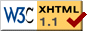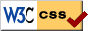# (A072444 - A072447)

In June 2002 I submitted the following four 'Sub Power Set Sequences' to Neil Sloane's On-Line Encyclopedia of Integer Sequences with the A-numbers: A072444, A072445, A072446 and A072447.

## Definitions

Consider the subsets S of the power set P({1,2,..,n}) that are closed under the following property:

• If X and Y are elements of S and X and Y have a non-empty intersection, then the union of X and Y is an element of S.

For example, {{1},{2},{1,2},{1,3},{1,2,3}} is such a subset, but {{1},{2},{1,2},{1,3}} is not.
We use the convention that all single element sets {j} are elements of S.

We ask the question: "For a given n, how many sets S are there?"

We can expect this number to grow double-exponential with n as there are potentially 2^(2^n) subsets of P({1,...,n}). The data below indicates that this is indeed probably the case.

We make two choices when counting the sets S:

1. Do we force the maximal subset {1,2,...,n} to be part of S?
2. How do we count sets that are permutations of other sets? That is: are {{1},{2},{3},{1,2}} and {{1},{2},{3},{1,3}} different sets or not?

By varying these options we get the following four sequences.

## Sub Power Sets, modulo permutations (A072444): 1, 2, 6, 47, 3095, 26015236,...

Let a(n) be the number of subsets S of the power set P({1,2,...,n}) such that

• {1}, {2},..., {n} are all elements of S
• If X and Y are elements of S and X and Y have a non-empty intersection, then the union of X and Y is an element of S
• The sets S are counted modulo permutations on the elements 1,2,...,n.

The first 6 values are as follows:

• a(1)=1, by {{1}}
• a(2)=2, by the sets {{1},{2}} and {{1},{2},{1,2}}
• a(3)=6, according to the 6 sets
• {{1},{2},{3}}
• {{1},{2},{3},{1,2}}
• {{1},{2},{3},{1,2,3}}
• {{1},{2},{3},{1,2},{1,2,3}}
• {{1},{2},{3},{1,2},{1,3},{1,2,3}}
• {{1},{2},{3},{1,2},{1,3},{2,3},{1,2,3}}
• Here you can view the lists that show that a(4)=47 and a(5)=3095.
(Note the shorthand where {1,2,12} stands for {{1},{2},{1,2}}, et cetera.)
• The fact that a(6)=26015236 was proven with the values b(1),...,b(6) below.

## Sub Power Sets with maximum subset, modulo permutations (A072445): 1, 1, 4, 40, 3044, 26012090,...

Let b(n) be the number of subsets S of the power set P({1,2,...,n}) such that

• {1}, {2},..., {n} are all elements of S
• {1,2,...,n} is an element of S
• If X and Y are elements of S and X and Y have a non-empty intersection, then the union of X and Y is an element of S
• The sets S are counted modulo permutations on the elements 1,2,...,n.

The first 6 values are as follows:

• b(1)=1, by {{1}}
• b(2)=1, by {{1},{2},{1,2}}
• b(3)=4 according to the 4 sets
• {{1},{2},{3},{1,2,3}}
• {{1},{2},{3},{1,2},{1,2,3}}
• {{1},{2},{3},{1,2},{1,3},{1,2,3}}
• {{1},{2},{3},{1,2},{1,3},{2,3},{1,2,3}}
• Here you can view the lists that show that b(4)=40 and b(5)=3044.
(Note the shorthand where {1,2,12} stands for {{1},{2},{1,2}}, et cetera.)
• The fact that b(6)=26012090 was proven by a brute force search that took several days on a Pentium II computer.

## Sub Power Sets (A072446): 1, 2, 12, 420, 254076, 17199454920,...

Let c(n) be the number of subsets S of the power set P({1,2,...,n}) such that

• {1}, {2},..., {n} are all elements of S
• If X and Y are elements of S and X and Y have a non-empty intersection, then the union of X and Y is an element of S

The first 5 values are as follows:

• c(1)=1, by {{1}}
• c(2)=2, by {{1},{2}} and {{1},{2},{1,2}}
• c(3)=12 according to the 12 sets
• {{1},{2},{3}}
• {{1},{2},{3},{1,2}}
• {{1},{2},{3},{1,3}}
• {{1},{2},{3},{2,3}}
• {{1},{2},{3},{1,2,3}}
• {{1},{2},{3},{1,2},{1,2,3}}
• {{1},{2},{3},{1,3},{1,2,3}}
• {{1},{2},{3},{2,3},{1,2,3}}
• {{1},{2},{3},{1,2},{1,3},{1,2,3}}
• {{1},{2},{3},{1,2},{2,3},{1,2,3}}
• {{1},{2},{3},{1,3},{2,3},{1,2,3}}
• {{1},{2},{3},{1,2},{1,3},{2,3},{1,2,3}}
• Here you can view the list that shows that c(4)=420
(Note the shorthand where {1,2,12} stands for {{1},{2},{1,2}}, et cetera.)
• c(5)=254076
• The fact that c(6)=17199454920 took a week to compute on a Pentium II.

## Sub Power Sets with maximum subset (A072447): 1, 1, 8, 378, 252000, 17197930224,...

Let d(n) be the number of subsets S of the power set P({1,2,...,n}) such that

• {1}, {2},..., {n} are all elements of S
• {1,2,...,n} is an element of S
• If X and Y are elements of S and X and Y have a non-empty intersection, then the union of X and Y is an element of S

The first 5 values are as follows:

• d(1)=1, by {{1}}
• d(2)=1, by {{1},{2},{1,2}}
• d(3)=8 according to the 8 sets
• {{1},{2},{3},{1,2,3}}
• {{1},{2},{3},{1,2},{1,2,3}}
• {{1},{2},{3},{1,3},{1,2,3}}
• {{1},{2},{3},{2,3},{1,2,3}}
• {{1},{2},{3},{1,2},{1,3},{1,2,3}}
• {{1},{2},{3},{1,2},{2,3},{1,2,3}}
• {{1},{2},{3},{1,3},{2,3},{1,2,3}}
• {{1},{2},{3},{1,2},{1,3},{2,3},{1,2,3}}
• Here you can view the list that shows that d(4)=378
(Note the shorthand where {1,2,12} stands for {{1},{2},{1,2}}, et cetera.)
• d(5)=252000
• The determination of the value d(6)=17197930224 was part of the computation of c(6).last update: August 2006 by vandam@cs